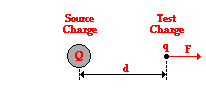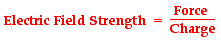# Define the term electric field intensity. Explain the terms source charge and test charge.

13 years ago

Dear anuj,

Electric field strength is a vector quantity; it has both magnitude and direction. The magnitude of the electric field strength is defined in terms of how it is measured. Let's suppose that an electric chargecan be denoted by the symbol Q. This electric charge creates an electric field; since Q is the source of the electric field, we will refer to it as the source charge. The strength of the source charge's electric field could be measured by any other charge placed somewhere in its surroundings. The charge that is used to measure the electric field strength is referred to as a test charge since it is used to test the field strength. The test charge has a quantity of charge denoted by the symbol q. When placed within the electric field, the test charge will experience an electric force - either attractive or repulsive. As is usually the case, this force will be denoted by the symbol F. The magnitude of the electric field is simply defined as the force per charge on the test charge.All the best.